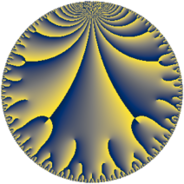# Properties

 Label 95.11.jLevel $95$ Weight $11$ Character orbit 95.j Rep. character $\chi_{95}(31,\cdot)$ Character field $\Q(\zeta_{6})$ Dimension $136$ Newform subspaces $1$ Sturm bound $110$ Trace bound $0$

# Related objects

## Defining parameters

 Level: $$N$$ $$=$$ $$95 = 5 \cdot 19$$ Weight: $$k$$ $$=$$ $$11$$ Character orbit: $$[\chi]$$ $$=$$ 95.j (of order $$6$$ and degree $$2$$) Character conductor: $$\operatorname{cond}(\chi)$$ $$=$$ $$19$$ Character field: $$\Q(\zeta_{6})$$ Newform subspaces: $$1$$ Sturm bound: $$110$$ Trace bound: $$0$$

## Dimensions

The following table gives the dimensions of various subspaces of $$M_{11}(95, [\chi])$$.

Total New Old
Modular forms 204 136 68
Cusp forms 196 136 60
Eisenstein series 8 0 8

## Trace form

 $$136 q - 132 q^{3} + 37474 q^{4} - 1022 q^{6} - 76620 q^{7} + 1448988 q^{9} + O(q^{10})$$ $$136 q - 132 q^{3} + 37474 q^{4} - 1022 q^{6} - 76620 q^{7} + 1448988 q^{9} + 93750 q^{10} + 418144 q^{11} - 1256574 q^{13} + 612480 q^{14} - 20575630 q^{16} + 3635834 q^{17} + 10572814 q^{19} + 8494308 q^{21} - 31311360 q^{22} - 11728554 q^{23} - 1627348 q^{24} - 132812500 q^{25} - 5280948 q^{26} - 81401852 q^{28} - 36928518 q^{29} + 79750000 q^{30} + 196574250 q^{32} + 359004150 q^{33} + 205341462 q^{34} + 19937500 q^{35} - 1128154804 q^{36} + 1584974 q^{38} + 224945180 q^{39} + 96000000 q^{40} + 1170402744 q^{41} + 173440898 q^{42} - 390292220 q^{43} - 49120052 q^{44} - 148625000 q^{45} - 445097680 q^{47} + 362033562 q^{48} + 3958185060 q^{49} + 995976516 q^{51} + 7766334 q^{52} + 815693040 q^{53} + 2049344062 q^{54} + 3831676392 q^{57} + 3983452220 q^{58} + 2842939668 q^{59} - 1389656250 q^{60} + 1929307508 q^{61} - 2885441744 q^{62} - 5022307122 q^{63} - 29425336368 q^{64} + 2939512012 q^{66} + 1891858296 q^{67} + 18554561860 q^{68} - 810562500 q^{70} - 8973340848 q^{71} + 7541812308 q^{72} + 4947578188 q^{73} - 23389998076 q^{74} + 15724739728 q^{76} - 5682818404 q^{77} - 10507817400 q^{78} + 16066927146 q^{79} + 3604750000 q^{80} - 43776877608 q^{81} - 13564413998 q^{82} + 2628386824 q^{83} - 2399750000 q^{85} + 38885030448 q^{86} + 12840668220 q^{87} - 35640122064 q^{89} + 3198281250 q^{90} + 4174912572 q^{91} - 23134882520 q^{92} - 36221884016 q^{93} - 1613500000 q^{95} + 13864395128 q^{96} - 23558943450 q^{97} + 31730410686 q^{98} - 8878413234 q^{99} + O(q^{100})$$

## Decomposition of $$S_{11}^{\mathrm{new}}(95, [\chi])$$ into newform subspaces

Label Dim $A$ Field CM Traces $q$-expansion
$a_{2}$ $a_{3}$ $a_{5}$ $a_{7}$
95.11.j.a $136$ $60.359$ None $$0$$ $$-132$$ $$0$$ $$-76620$$

## Decomposition of $$S_{11}^{\mathrm{old}}(95, [\chi])$$ into lower level spaces

$$S_{11}^{\mathrm{old}}(95, [\chi]) \cong$$ $$S_{11}^{\mathrm{new}}(19, [\chi])$$$$^{\oplus 2}$$